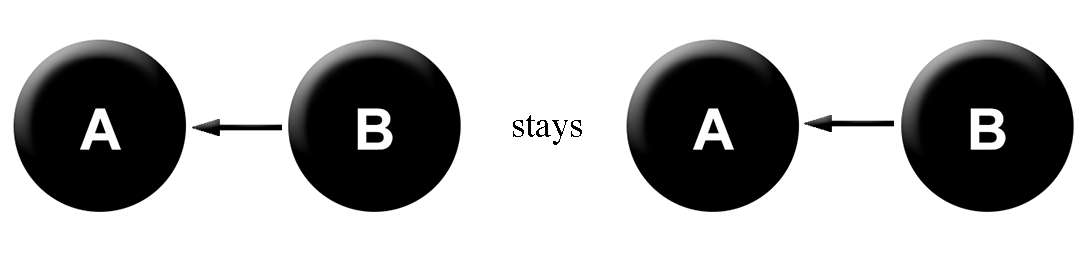# Causal Intervention

Previous post: Causal arrows

Let’s quickly review the last post. A causal diagram for two variables A and B tells us how to factor the joint probability distribution P(A & B). The rule we use is that for each variable, we calculate its probability conditional upon all of its parent nodes. This can easily be generalized to any number of variables.

Quick exercises: See if you understand why the following are true.

1. If the causal relationships between three variables A, B, and C are:Then P(A & B & C) = P(A) · P(B | A) · P(C | B).

2. If the causal relationships are:Then P(A & B & ~C) = P(A | B) · P(B) · P(~C | B).

3. If the causal relationships are:Then P(~A & ~B & C) = P(~A) · P(~B | ~A & C) · P(C)

Got it? Then you’re ready to move on!

***

Two people are debating a causal question. One of them says that the rain causes the sidewalk to get wet. The other one says that the sidewalk being wet causes the rain. We can express their debate as:We’ve already seen that the probability distributions that correspond to these causal models are empirically indistinguishable. So how do we tell who’s right?

Easy! We go outside with a bucket of water and splash it on the sidewalk. Then we check and see if it’s raining. Another day, we apply a high-powered blow-drier to the sidewalk and check if it’s raining.

We repeat this a bunch of times at random intervals, and see if we find that splashing the sidewalk makes it any more likely to rain than blow-drying the sidewalk. If so, then we know that sidewalk-wetness causes rain, not the other way around.

This is the process of intervention. When we intervene on a variable, we set it to some desired value and see what happens. Let’s express this with our diagrams.

When we splash the sidewalk with water, what we are in essence doing is setting the variable B (“The sidewalk is wet”) to true. And when we blow-dry the sidewalk, we are setting the variable B to false. Since we are now the complete determinant of the value of B, all causal arrows pointing towards B must be erased. So:andAnd now our intervened-upon distributions are empirically distinguishable!

The person who thinks that sidewalk-wetness causes rain expects to find a probabilistic dependence between A and B when we intervene. In particular, they expect that it will be more likely to rain when you splash the sidewalk than when you blow-dry it.

And the person who thinks that rain causes sidewalk-wetness expects to find no probabilistic dependence between A and B. They’ll expect that it is equally likely to be raining if you’re splashing the sidewalk as if you’re blow-drying it.

***

This is how to determine the direction of causal arrows using causal models. The key insight here is that a causal model tells you what happens when you perform interventions.

The rule is: Causal intervention on a variable X is represented by erasing all incoming arrows to X and setting its value to its intervened value.

I’ll introduce one last concept here before we move on to the next post: the causal conditional probability.

In our previous example, we talked about the probability that it rains, given that you splash the sidewalk. This is clearly different than the probability that it rains, given that the sidewalk is wet. So we give it a new name.

Normal conditional probability = P(A | B) = probability that it rains given that the sidewalk is wet

Causal conditional probability = P(A | do B) = probability that it rains given that you splash the sidewalk.

The causal conditional probability of A given B, is just the probability of A given that you intervene on B and set it to “True”. And P(A | do ~B) is the probability of A given that you intervene on B and set it to “False”.
If we find that P(A | do B) = P(A | do ~B), then we have ruled out.

Previous: Causal arrows